• 1.2 数字滤波器的系统函数和差分方程 1.3 数字滤波器结构的表示 1.4 数字滤波器的分类 2.1 IIR滤波器与FIR滤波器的分析比较 2.2 FIR滤波器的原理 3 FIR滤波器的仿真步骤 二、源代码 %--------------...
一、简介
1 设计原理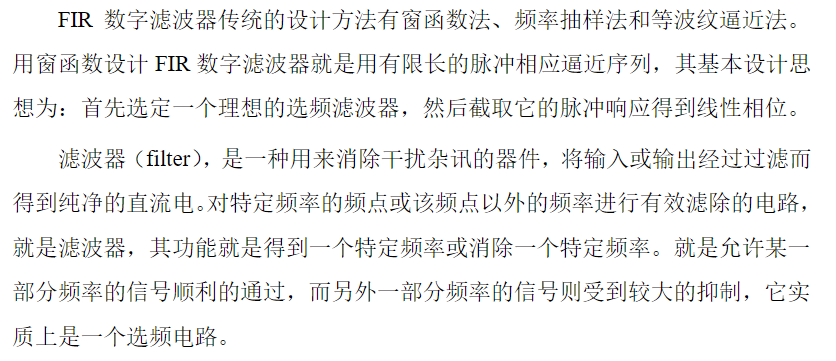1.1 滤波器概念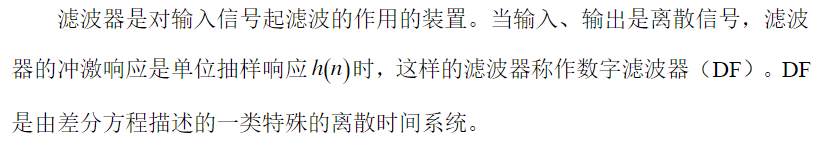1.2 数字滤波器的系统函数和差分方程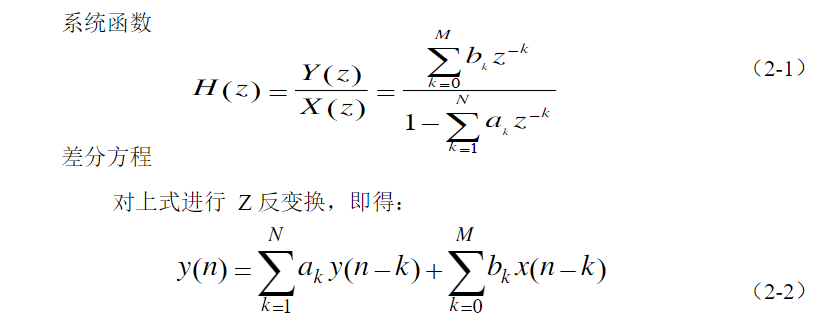1.3 数字滤波器结构的表示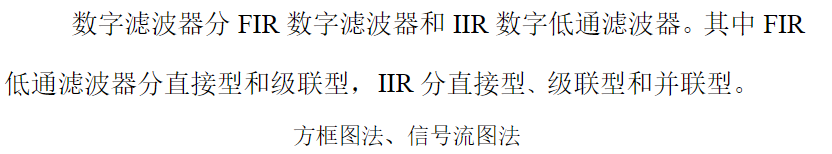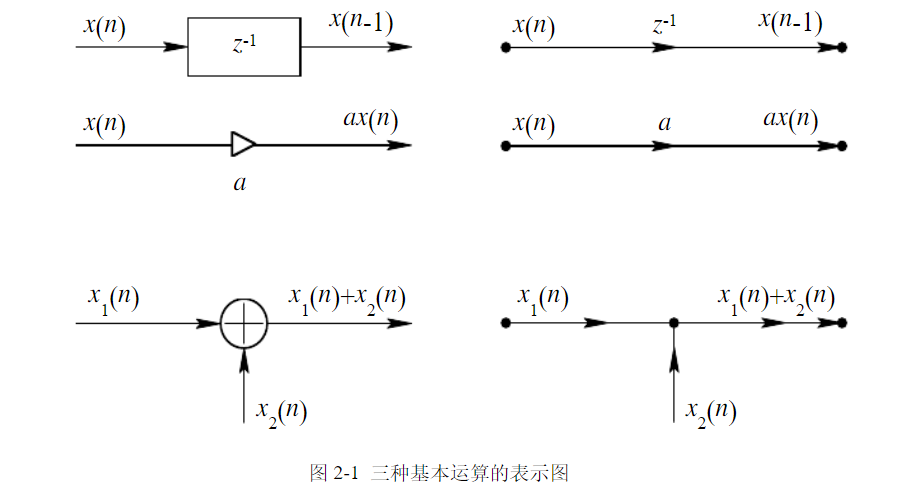1.4 数字滤波器的分类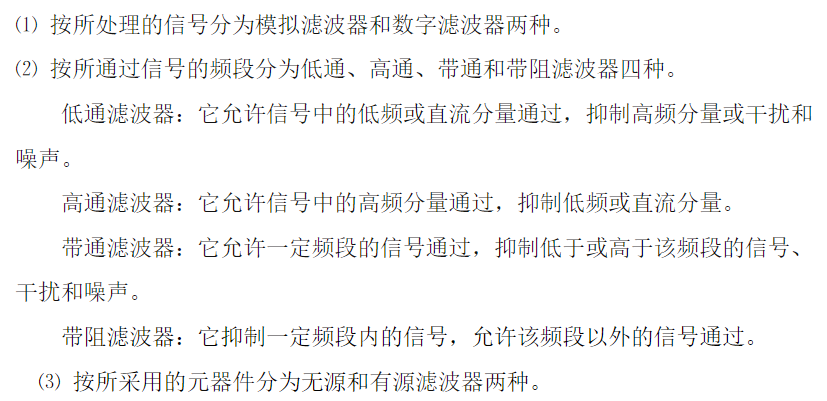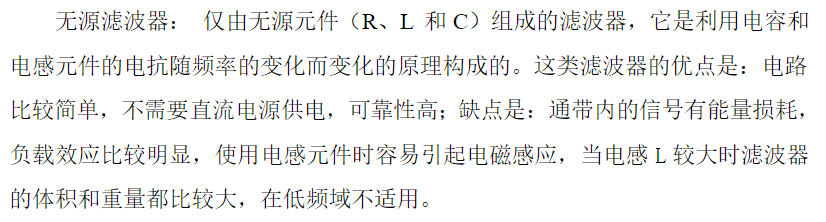2.1  IIR滤波器与FIR滤波器的分析比较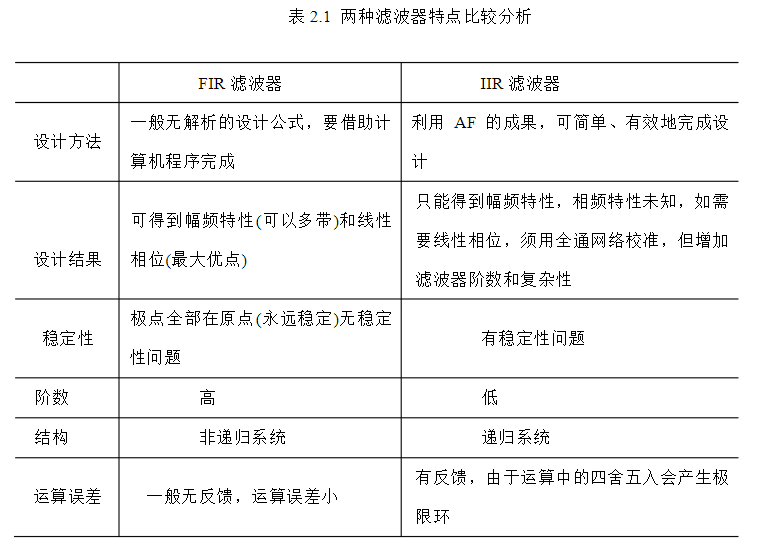2.2 FIR滤波器的原理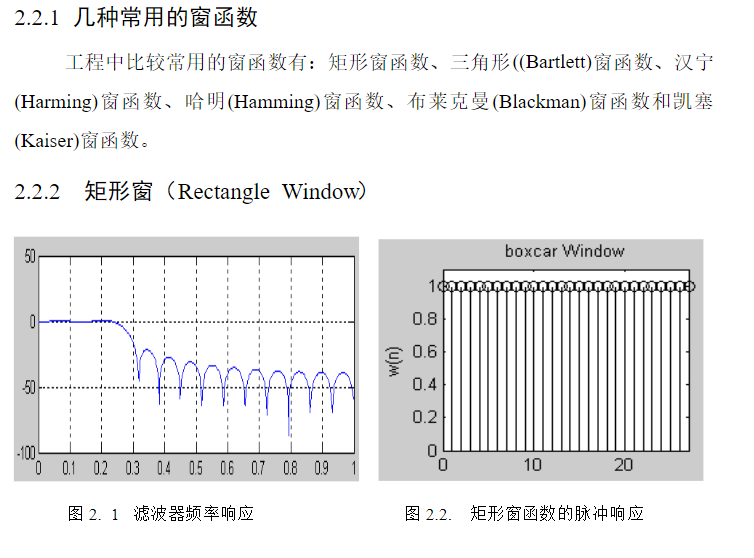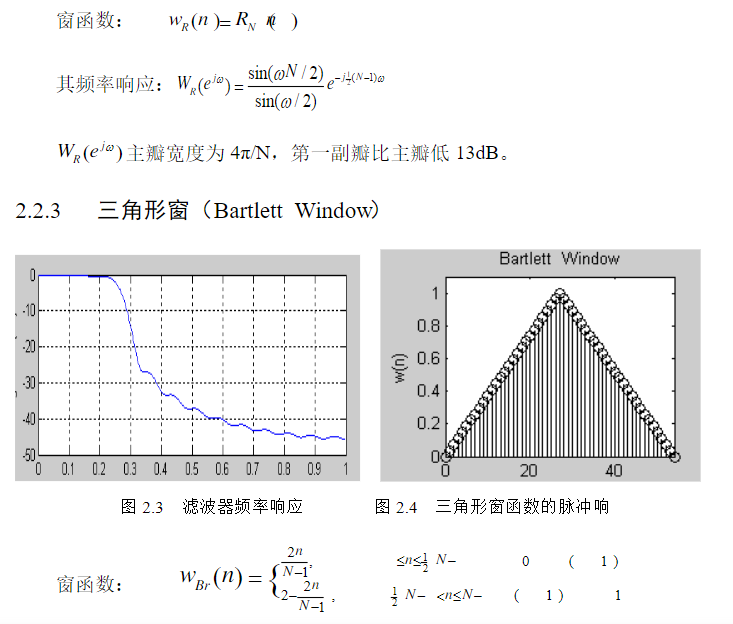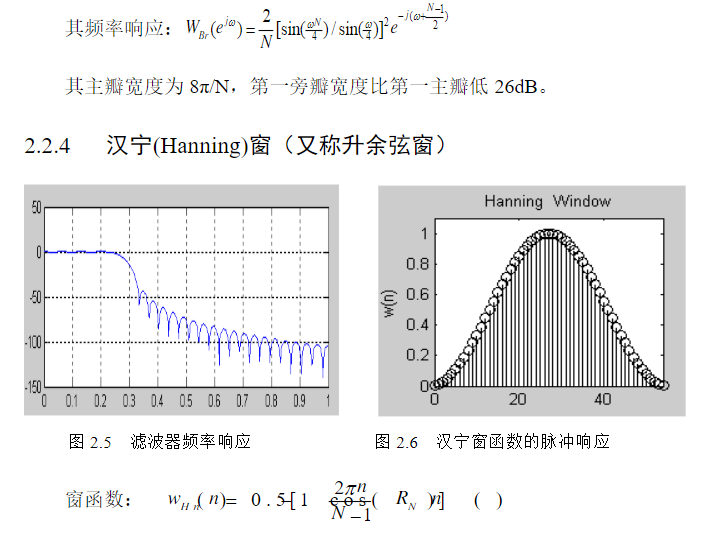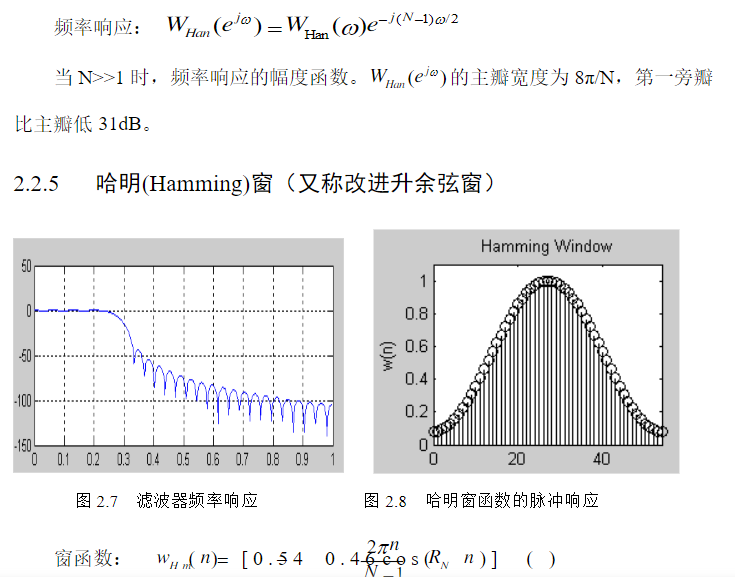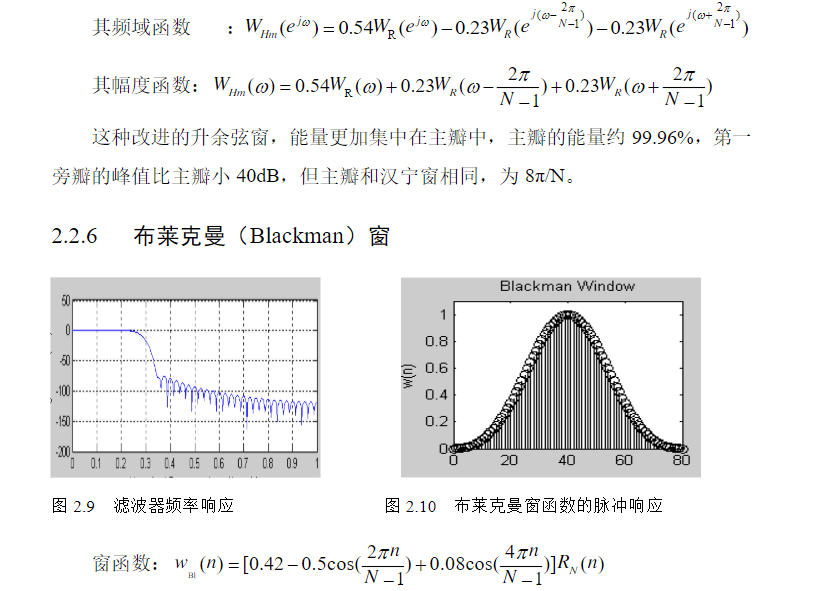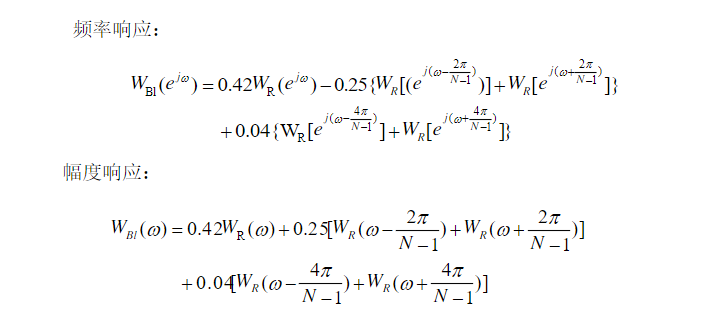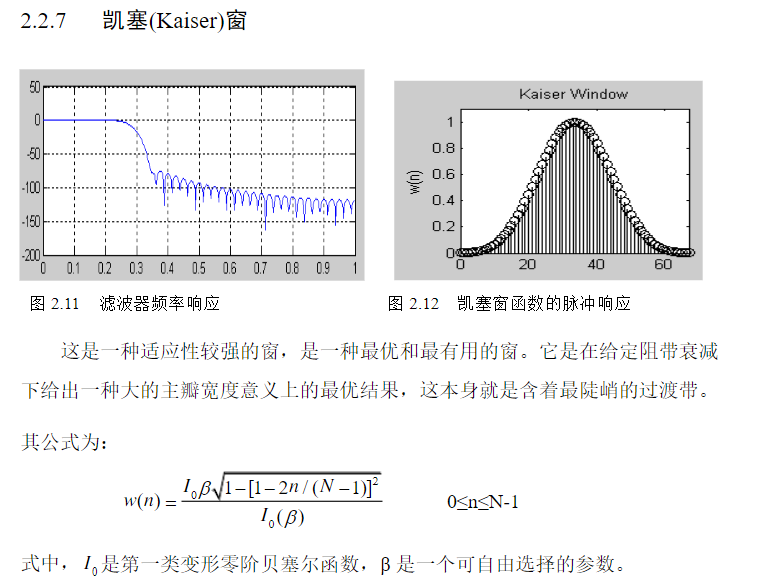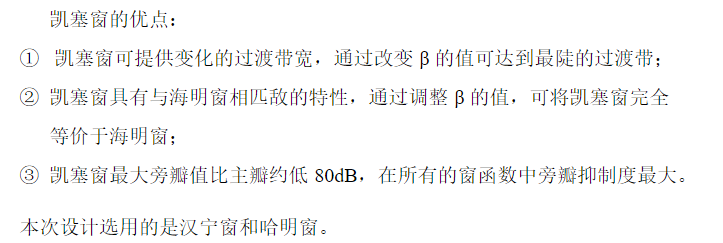3 FIR滤波器的仿真步骤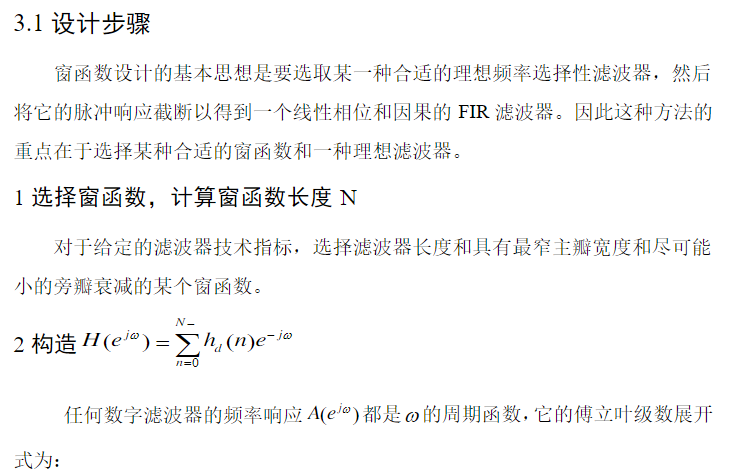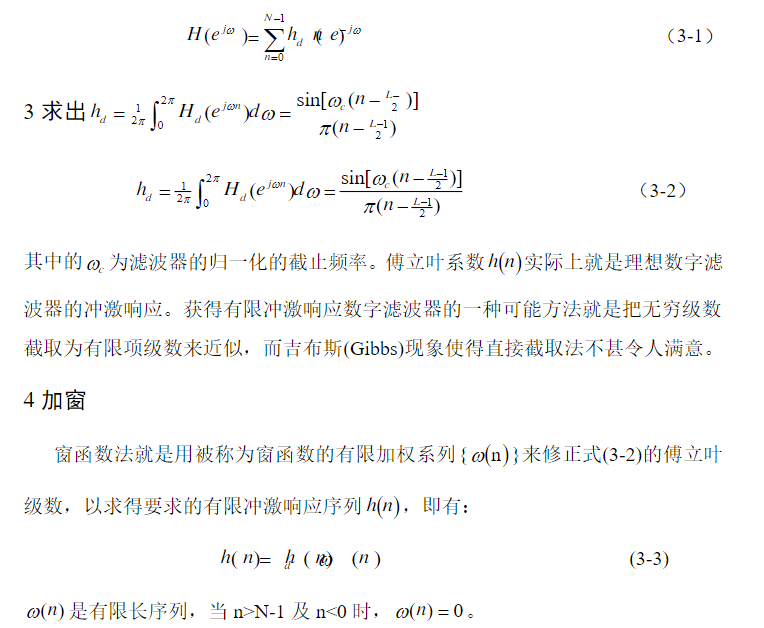二、源代码
%--------------------------------------------------------------------------
%利用kaiser窗设计低通滤波器m文件
%默认输入参数：   N=64
%                beta=5.568
%                wc=0.5pi
%输出参数：      通带边界（wp）
%               阻带边界（ws）
%               通带波纹
%               阻带衰减
%--------------------------------------------------------------------------
function varargout = lpfilter(varargin)
% LPFILTER M-file for lpfilter.fig
%      LPFILTER, by itself, creates a new LPFILTER or raises the existing
%      singleton*.
%
%      H = LPFILTER returns the handle to a new LPFILTER or the handle to
%      the existing singleton*.
%
%      LPFILTER('CALLBACK',hObject,eventData,handles,...) calls the local
%      function named CALLBACK in LPFILTER.M with the given input arguments.
%
%      LPFILTER('Property','Value',...) creates a new LPFILTER or raises the
%      existing singleton*.  Starting from the left, property value pairs are
%      applied to the GUI before lpfilter_OpeningFunction gets called.  An
%      unrecognized property name or invalid value makes property application
%      stop.  All inputs are passed to lpfilter_OpeningFcn via varargin.
%
%      *See GUI Options on GUIDE's Tools menu.  Choose "GUI allows only one
%      instance to run (singleton)".
%

% Edit the above text to modify the response to help lpfilter

% Begin initialization code - DO NOT EDIT
gui_Singleton = 1;
gui_State = struct('gui_Name',       mfilename, ...
'gui_Singleton',  gui_Singleton, ...
'gui_OpeningFcn', @lpfilter_OpeningFcn, ...
'gui_OutputFcn',  @lpfilter_OutputFcn, ...
'gui_LayoutFcn',  [] , ...
'gui_Callback',   []);
if nargin & isstr(varargin{1})
gui_State.gui_Callback = str2func(varargin{1});
end

if nargout
[varargout{1:nargout}] = gui_mainfcn(gui_State, varargin{:});
else
gui_mainfcn(gui_State, varargin{:});
end
% End initialization code - DO NOT EDIT

% --- Executes just before lpfilter is made visible.
function lpfilter_OpeningFcn(hObject, eventdata, handles, varargin)
% This function has no output args, see OutputFcn.
% hObject    handle to figure
% eventdata  reserved - to be defined in a future version of MATLAB
% handles    structure with handles and user data (see GUIDATA)
% varargin   command line arguments to lpfilter (see VARARGIN)

% Choose default command line output for lpfilter
handles.output = hObject;

% Update handles structure
guidata(hObject, handles);

% UIWAIT makes lpfilter wait for user response (see UIRESUME)
% uiwait(handles.figure1);
%--------------------------------------------------------------------------
%设置滤波器长度，beta和截止频率编辑框的初始值
%设置窗体的标题
set(handles.figure1,'name','FIR低通滤波器');
set(handles.edit_N,'string','64');
set(handles.edit_beta,'string','5.568');
set(handles.edit_fc,'string','0.5');
%--------------------------------------------------------------------------
%由设置的初始值求取滤波器的理想单位脉冲响应
handles.wc=0.5*pi;
handles.M=64;
handles.hd=ideal_lp(handles.wc,handles.M);
%--------------------------------------------------------------------------
%绘制滤波器的理想单位脉冲响应
axes(handles.axes_hd);
n=[0:1:handles.M-1];
plot(n,handles.hd);
%%%%%%%%%%%%%%%%%%%%%%%%%%%%%%%%%%%%%%%%%%%%%%%%%%%55

title('滤波器理想脉冲响应');
axis([0 handles.M-1 min(handles.hd) max(handles.hd)+0.01]);
xlabel('n');
ylabel('hd(n)');
grid on;
guidata(hObject,handles);

% --- Executes on button press in plot.
function plot_Callback(hObject, eventdata, handles)
% hObject    handle to plot (see GCBO)
% eventdata  reserved - to be defined in a future version of MATLAB
% handles    structure with handles and user data (see GUIDATA)
%--------------------------------------------------------------------------
%从编辑框获取滤波器长度，beta和截止频率的值
handles.M = str2double(get(handles.edit_N,'String'));
handles.beta = str2double(get(handles.edit_beta,'String'));
handles.wc = str2double(get(handles.edit_fc,'String'))*pi;
%求取滤波器的理想单位脉冲响应
handles.hd=ideal_lp(handles.wc,handles.M);
%绘制滤波器的理想单位脉冲响应
axes(handles.axes_hd);
plot(0,0);
n=[0:1:handles.M-1];
plot(n,handles.hd);
title('滤波器理想脉冲响应');
axis([0 handles.M-1 min(handles.hd) max(handles.hd)]);
xlabel('n');
ylabel('hd(n)');
grid on;
%--------------------------------------------------------------------------
%求取kaiser窗函数
handles.w_kai=(kaiser(handles.M,handles.beta))';
%绘制kaiser窗函数
axes(handles.axes_wkaiser);
plot(n,handles.w_kai);
title('kaiser窗');
axis([0 handles.M-1 min(handles.w_kai) max(handles.w_kai)]);
xlabel('n');
ylabel('w(n)');
grid on;
%--------------------------------------------------------------------------
%求取滤波器的实际单位脉冲响应
handles.h=handles.hd.*handles.w_kai;
%绘制滤波器的实际单位脉冲响应
axes(handles.axes_h);
plot(n,handles.h);
title('滤波器实际脉冲响应');
axis([0 handles.M-1 min(handles.h) max(handles.h)]);
xlabel('n');
ylabel('h(n)');
grid on;
%--------------------------------------------------------------------------
%求取滤波器的频率特性，并绘制幅频特性（转换到0—pi）
[H,w] = freqz(handles.h,,handles.M*6,'whole');
% freqz(handles.h,,handles.M*6);
H=(H(1:1:handles.M*3))';
w=(w(1:1:handles.M*3))';
mag=abs(H);
db=20*log10((mag+eps)/max(mag));
% pha=angle(H);
%绘制幅频特性（转换到0—pi）
axes(handles.axes_lp);
plot(w/pi,db);
title('FIR滤波器的幅频特性（0-pi）');
axis([0 1 min(db) max(db)]);
xlabel('Frequency in pi units');
ylabel('Decibels');
grid on;
guidata(hObject,handles);
%--------------------------------------------------------------------------
%由求得幅频特性计算通带边界，阻带边界，通带纹波和阻带衰减
%由beta值计算阻带衰减As
if handles.beta<4.5513
As=fzero(@myfun,20,[],handles.beta)+21;
else
As=handles.beta/0.1102+8.7;
end
%计算通带边界wp，阻带边界ws
w_w=(As-7.95)/((handles.M-1)*14.36)*2;
ws=0.5*(w_w+2*handles.wc/pi);
wp=0.5*(2*handles.wc/pi-w_w);
% mag=(mag+eps)/max(mag);
%计算通带纹波rp
% deta_w=2*pi/(handles.M*6);
% w_temp=mag(floor(ws/deta_w));
% mag_temp=abs(mag(1:floor(ws/deta_w))-w_temp);
rp=20*log10(max(mag));
%--------------------------------------------------------------------------
%在相应的编辑框中显示通带边界，阻带边界，通带纹波和阻带衰减
str=sprintf('%.2f',wp);
set(handles.edit_wp,'string',str);
str=sprintf('%.2f',ws);
set(handles.edit_ws,'string',str);
str=sprintf('%.4f',rp);
set(handles.edit_rp,'string',str);
str=sprintf('%.2f',-As);
set(handles.edit_As,'string',str);
%--------------------------------------------------------------------------
%退出程序
function exit_Callback(hObject, eventdata, handles)
% hObject    handle to exit (see GCBO)
% eventdata  reserved - to be defined in a future version of MATLAB
% handles    structure with handles and user data (see GUIDATA)
close(handles.figure1);

%--------------------------------------------------------------------------
%自定义函数，计算理想低通滤波器的单位脉冲响应
function hd=ideal_lp(wc,M);
alpha=(M-1)/2;
n = [0:1:(M-1)];
m=n-alpha+eps;
hd=sin(wc*m)./(pi*m);
% --- Outputs from this function are returned to the command line.
function varargout = lpfilter_OutputFcn(hObject, eventdata, handles)
% varargout  cell array for returning output args (see VARARGOUT);
% hObject    handle to figure
% eventdata  reserved - to be defined in a future version of MATLAB
% handles    structure with handles and user data (see GUIDATA)

% Get default command line output from handles structure
varargout{1} = handles.output;

% --- Executes during object creation, after setting all properties.
function edit_N_CreateFcn(hObject, eventdata, handles)
% hObject    handle to edit_N (see GCBO)
% eventdata  reserved - to be defined in a future version of MATLAB
% handles    empty - handles not created until after all CreateFcns called

% Hint: edit controls usually have a white background on Windows.
%       See ISPC and COMPUTER.
if ispc
set(hObject,'BackgroundColor','white');
else
set(hObject,'BackgroundColor',get(0,'defaultUicontrolBackgroundColor'));
end

function edit_N_Callback(hObject, eventdata, handles)
% hObject    handle to edit_N (see GCBO)
% eventdata  reserved - to be defined in a future version of MATLAB
% handles    structure with handles and user data (see GUIDATA)

% Hints: get(hObject,'String') returns contents of edit_N as text
%        str2double(get(hObject,'String')) returns contents of edit_N as a double

% --- Executes during object creation, after setting all properties.
function edit_beta_CreateFcn(hObject, eventdata, handles)
% hObject    handle to edit_beta (see GCBO)
% eventdata  reserved - to be defined in a future version of MATLAB
% handles    empty - handles not created until after all CreateFcns called

% Hint: edit controls usually have a white background on Windows.
%       See ISPC and COMPUTER.
if ispc
set(hObject,'BackgroundColor','white');
else
set(hObject,'BackgroundColor',get(0,'defaultUicontrolBackgroundColor'));
end

function edit_beta_Callback(hObject, eventdata, handles)
% hObject    handle to edit_beta (see GCBO)
% eventdata  reserved - to be defined in a future version of MATLAB
% handles    structure with handles and user data (see GUIDATA)

% Hints: get(hObject,'String') returns contents of edit_beta as text
%        str2double(get(hObject,'String')) returns contents of edit_beta as a double

三、运行结果四、备注
版本：2014a


展开全文• ## 数字滤波器的设计

千次阅读 2020-10-31 14:17:03
FT、LT、ZT之间的（映射）关系零、极点分布如何影响频率响应从均值滤波看滤波器及系统函数时域表达式频率表达式零极点分布图频率响应分析FIR和IIR滤波器的比较性能上结构上设计工具上效果上FIR滤波器的设计基本设计...
文章目录先导知识时频域互换什么是滤波器？如何设计滤波器？FT、LT、ZT之间的（映射）关系零、极点分布如何影响频率响应从均值滤波看滤波器及系统函数时域表达式频率表达式零极点分布图频率响应分析FIR和IIR滤波器的比较性能上结构上设计工具上效果上FIR滤波器的设计基本设计思路窗函数法频率采样法响应最优法最小二乘法切比雪夫等波纹最佳逼近法FIR数字滤波器设计总结IIR滤波器设计基本设计思路模拟滤波器的设计巴特沃斯滤波器如何根据指标求出滤波器阶数N低通巴特沃斯滤波器设计步骤设计低通巴特沃斯滤波器实例切比雪夫I型滤波器椭圆滤波器高通、带通和带阻滤波器的设计归一化低通滤波器低通到高通的频率转换低通到带通的频率转换低通到带阻的频率转换模拟滤波器一般设计步骤书上的频率变换映射关系模拟低通到高通设计实例模拟低通到带通设计实例模拟低通到带阻从模拟到数字的转换方法脉冲响应不变法基本思路推导过程双线性变换法基本思路转换性能分析IIR滤波器设计实例IIR数字滤波器设计总结

Author🚹：CofCai
personal page🏠
CSDN page🏠
QQ🔭：1664866311

说明：本文并非全部原创，是在网上学习各位前辈的文章后写的学习总结，文中有些图片和代码使用了网上的文章，若侵比删。所参考文章的链接已在文中给出！
先导知识
时频域互换
从时域我们很难看出信号包含那些频率成分，也很难去掉信号中某些特定的成分。如果能将信号表达成不同频率成分之和，那么不就很容易看出该信号包含那些频率成分了吗。此时，就需要傅里叶级数（周期信号）、傅里叶变换（非周期信号）等。对于离散信号，需要DTFT（离散时间傅里叶变换）、DFS（离散傅里叶级数、离散周期信号）、DFT（离散傅里叶变换、离散非周期信号）等。

连续（模拟）信号

FS
将周期连续信号变换到频域。

FT
将非周期连续信号变换到频域。

LT
变换到复平面，及s平面。

离散（数字）信号

DTFT
将数字信号变换到频域，此时频域是连续的。

DFS
将周期数字信号变换到频域。

DFT
将数字信号变换到频域，此时频域也是离散的。

ZT
变换到z平面。

什么是滤波器？
滤波器就是滤掉干扰信息（噪声），保留有用信息。
下面分别是按滤掉噪声频率的几类滤波器的幅频曲线图：设计常规滤波器的时候，我们一般采用另外一种分类，FIR（Finite Impulse Response）和IIR（Infinite Impulse Response）filter，即有限脉冲响应滤波器和无限脉冲响应滤波器。
理想脉冲信号，其傅里叶变换恒为1，也就时包含了所有频率分量，是一个理想的测试信号，能够激发出所有单位频率分量的响应，因此理想脉冲信号的响应，就代表了系统的特性。
滤波器也可以看成一个系统，如果用一个理想脉冲信号激励，就会有输出，我们把输出个数有限的称为有限脉冲响应滤波器（FIR）；输出无限多的称为无限脉冲响应滤波器（IIR）。
滤波器分为模拟滤波器和数字滤波器。模拟滤波器的理论和设计方法已经非常成熟，可以通过模拟滤波器间接设计数字滤波器。
如何设计滤波器？
就如第一幅图一样，我们可以通过其幅频曲线得到其截止频率、通带情况。那么怎么获得幅频曲线图呢？那就是通过系统函数。
$H(j\omega)=\left|H(j\omega)\right|e^{\theta(\omega)}$
$|H(j\omega)|$就是其幅频特性曲线，$\theta(\omega)$就是其相频特性曲线。
如何得到系统函数:
由系统的特性分析得到微分方程或差分方程，再拉普拉斯变换或z变换。
FT、LT、ZT之间的（映射）关系
LT是对连续FT的拓展，即从频率到复频域，$j{\omega}{\Longrightarrow}s={\delta}+j{\omega}$。
ZT是对离散FT的拓展，也可以看是对LT的再映射，即$z=e^{sT_s}$。
如何从DTFT到ZT，请看。简单说一下：
$DFT:X(j\omega)=\sum_{n=-\infin}^{+\infin}x(n)e^{j{\omega}nT_s}$
同FT到LT一样，为了满足绝对可和条件，乘上一个衰减因子$e^{{\delta}t}=e^{{\delta}nT_s}$(离散化)。
$X(j\omega)=\sum_{n=-\infin}^{+\infin}x(n)e^{j{\omega}nT_s}e^{{\delta}nT_s}\\ =\sum_{n=-\infin}^{+\infin}x(n)e^{-({\delta}+j{\omega})nT_s}$
令$z=e^{({\delta}+j{\omega})T_s}$，得到DFT的式子为：
$X(j{\omega})=\sum_{n=-\infin}^{+\infin}x(n)z^{-n}$
上式就是Z变换了。如何理解上面的规律？

需要明白幅频曲线自变量是频率$\omega$，而不是$s={\delta}+j{\omega}$。
$H(j{\omega})=|H(j{\omega})|e^{{\theta}(j{\omega})}=Num(j{\omega})/Den(j{\omega})$，其中的$|H(j\omega)|$就是幅频曲线、$\theta{（j\omega）}$就是相频响应。
s平面的虚轴（频率轴）被ZT映射到z平面的单位圆上。

因此，分析幅频响应时，频率轴在单位圆上，这也是为什么上面提到的规律都在说单位圆上的点怎么怎么样。

对于规律一，因为零点就在单位圆上，因此当频率为零点对应的频率时，$|H(j\omega)|=0$，因此幅值响应为零。
对于规律二，不在单位圆上的零点，单位圆上越靠近零点的频率，其$|H(j\omega)|$也就越小。
对于规律三，因为是极点，极点为0时即系统函数的分母为0，$|H(j\omega)|$很大。（至于为什么要指定是单位圆内部的极点呢？我猜是单位圆外部的极点使系统不稳定，因此不必讨论）零、极点分布如何影响频率响应
$H(z)=A\frac{\prod_{r=1}^M(1-c_rz^{-1})}{\prod_{r=1}^{N}(1-d_rz^{-1})} \\ |H(e^{j\omega})|=|A|\frac{\prod_{r=1}^M|z_r|}{\prod_{r=1}^N{|P_r|}} \\ \phi(\omega)=\omega(N-M)+\sum_{r=1}^{N}\alpha_r-\sum_{r=1}^{M}\beta_r$
即幅频响应等于所有的零点到$\omega$的模长的乘积除以所有的极点到$\omega$的模长的乘积；相频响应等于所有的零点与$\omega$形成的夹角之和减去所有的极点与$\omega$形成的夹角之和。
从均值滤波看滤波器及系统函数
时域表达式
$y(n)=\frac{1}{N}\sum_{k=0}^Nx(n-k)\\=\frac{1}{N}\left[x(n)+...+x(n-N+1)\right]$
$x(n)$是当前测量值，$x(n-N+1)$是前第N-1次测量值。
频率表达式
对均值滤波的时域表达式进行Z变换得：
$H(z)=\frac{1}{N}\sum_{k=0}^{N}z^{-N}\\=\frac{1}{N}\frac{1-z^{-N}}{1-z^{-1}}$
令$z=e^{j\omega}$得：
$H(e^{j\omega})=\frac{1}{N}\frac{1-e^{-j{\omega}N}}{1-e^{-j{\omega}}}\\=\frac{1}{N}e^{-j\frac{\omega(N-1)}{2}}\frac{\sin({\omega}N)/2}{\sin(\omega)/2}$
零极点分布图
$H(z)=\frac{1}{N}\frac{1-z^{-N}}{1-z^{-1}}\\=\frac{1}{N}\frac{z^{N}-1}{z^{N}-z^{N-1}}$
matlab代码：
NN = [3 6 12];

for i = 1:3
N = NN(i);
B = ones(1, N);
B = [B -1];
A = zeros(1, N-1);
A = [1 -1 A];
subplot(3, 1, i);
zplane(B, A);
xlabel(['N=', num2str(N)]);
end频率响应分析
python代码分析均值滤波频率响应，采样率为200Hz，阶数为7。
from scipy.optimize import newton
from scipy.signal import freqz, dimpulse, dstep
from math import sin, cos, sqrt, pi
import numpy as np
import matplotlib.pyplot as plt
import sys

#函数用于计算移动平均滤波器的截止频率
def get_filter_cutoff(N, **kwargs):
func = lambda w: sin(N*w/2) - N/sqrt(2) * sin(w/2)
deriv = lambda w: cos(N*w/2) * N/2 - N/sqrt(2) * cos(w/2) / 2
omega_0 = pi/N  # Starting condition: halfway the first period of sin
return newton(func, omega_0, deriv, **kwargs)

#设置采样率
sample_rate = 200 #Hz
N = 7

# 计算截止频率
w_c = get_filter_cutoff(N)
cutoff_freq = w_c * sample_rate / (2 * pi)

# 滤波器参数
b = np.ones(N)
a = np.array([N] + *(N-1))

#频率响应
w, h = freqz(b, a, worN=4096)
#转为频率
w *= sample_rate / (2 * pi)

#绘制波特图
plt.subplot(2, 1, 1)
plt.suptitle("Bode")
#转换为分贝
plt.plot(w, 20 * np.log10(abs(h)))
plt.ylabel('Magnitude [dB]')
plt.xlim(0, sample_rate / 2)
plt.ylim(-60, 10)
plt.axvline(cutoff_freq, color='red')
plt.axhline(-3.01, linewidth=0.8, color='black', linestyle=':')

# 相频响应
plt.subplot(2, 1, 2)
plt.plot(w, 180 * np.angle(h) / pi)
plt.xlabel('Frequency [Hz]')
plt.ylabel('Phase [°]')
plt.xlim(0, sample_rate / 2)
plt.ylim(-180, 90)
plt.yticks([-180, -135, -90, -45, 0, 45, 90])
plt.axvline(cutoff_freq, color='red')
plt.show()改变滤波器阶数，观察幅频响应（N=3，7，18）：可以看出，均值滤波器：

是一个低通滤波器
随着滤波器得阶数（长度）增加（求和的项越来越多），截止频率变小，通带变窄。滤波器的响应变慢，延迟变大。
是FIR滤波器

FIR和IIR滤波器的比较

稳定和线性相位特性是FIR滤波器最突出的优点，但阶数较高；IIR滤波器相位非线性，但阶数低。

性能上

IIR滤波器系统函数的极点可位于单位圆内的任何地方，因此零点和极点相结合，可用较低的阶数获得较高的选择性，所用的存储单元少，计算量小，经济效应高。但是这个高效率是以相位的非线性为代价的。
相反，FIR滤波器可以得到严格的线性相位，然而由于FIR滤波器系统函数的极点固定在原点，因而只能用较高的阶数达到较高的选择性。
对于同样的滤波器幅频特性指标，FIR滤波器所要求的阶数一般比IIR滤波器高5~10倍，信号延时也比较大。

结构上

IIR滤波器必须采用递归结构，极点位置必须在单位圆内，否则系统不稳定。另外，这种结构中由于运算过程中对序列的舍入处理，会引起寄生振荡。
FIR滤波器主要采用非递归结构，有限精度运算中不存在稳定性问题，运算误差引起的输出信号噪声功率也较小。此外，FIR滤波器可以采用FFT算法实现，速度大大提高。

设计工具上

IIR滤波器可以借助成熟的模拟滤波器设计理论及成果。
FIR滤波器计算带通和阻带衰减等仍无显示表达式，边界频率也不易精确控制。

效果上

IIR滤波器虽然设计简单，但主要是用于设计具有片段常数特性的选频型滤波器，比如低通、高通、带通及带阻等，往往脱离不了几种典型模拟滤波器的频响特性的约束。
而FIR滤波器要灵活得多，易于适应某些特殊的应用，比如只想滤掉特定的不连续的频率。

从上面可以看出，IIR和FIR滤波器各有所长，在实际运用当中应全面考虑再加以选择。比如，对于相位要求不高的场合，如语音通讯等可以选用IIR滤波器；对于图象信号处理等相位要求高的场合应采用FIR滤波器。
FIR滤波器的设计
如何快速设计一个FIR滤波器（一）
如何快速设计一个FIR滤波器（二）
基本设计思路
FIR滤波器的设计方法和IIR滤波器的设计方法有很大差别。FIR滤波器的设计任务是选择有限长度的$h(n)$，使频率响应函数$H(e^{j\omega})$满足技术指标要求。
下面介绍几种设计FIR滤波器的方法：窗函数法、频率采样法、响应最优法（切比雪夫等波纹逼近法、最小二乘法）。
窗函数法
窗函数的作用
加窗原理和频谱泄露 深入理解
FFT变换只能对有限长度的时域数据进行变换，因此，需要对时域信号进行信号截断。即使是周期信号，如果截断的时间长度不是周期的整数倍（周期截断），那么，截取后的信号将会存在泄漏。为了将这个泄漏误差减少到最小程度（注意我说是的减少，而不是消除），我们需要使用加权函数，也叫窗函数。加窗主要是为了使时域信号似乎更好地满足FFT处理的周期性要求，减少泄漏。
如下图所示：

若周期截断，则FFT频谱为单一谱线；
若为非周期截断，则频谱出现拖尾，如图中部所示，可以看出泄漏很严重。为了减少泄漏，给信号施加一个窗函数（如图中上部红色曲线所示），原始截断后的信号与这个窗函数相乘之后得到的信号为上面右侧的信号。可以看出，此时，信号的起始时刻和结束时刻幅值都为0，也就是说在这个时间长度内，信号为周期信号，但是只有一个周期。对这个信号做FFT分析，得到的频谱如下部右侧所示。相比较之前未加窗的频谱，可以看出，泄漏已明显改善，但并没有完全消除。因此，窗函数只能减少泄漏，不能消除泄漏。
基于窗函数法设计FIR滤波器，其基本步骤如下：

确定频域的响应函数$H_d(e^{j\omega})$，低通、高通、带通或者其它；
确定频域的响应函数$H_d(e^{j\omega})$的傅里叶逆变换，找到连续脉冲响应函数$h_d(t)$；
对连续脉冲响应函数$h_d(t)$按照一定的采样频率进行采样，获得离散信号$h_d(m)$；
选择合适的窗函数，对离散信号$h_d(m)$加窗，获得有限长度的脉冲响应$h(m)$。

MATLAB中基于窗函数法设计滤波器：
% h = fir1(n, Wn, ftype, window);
% n表示滤波器阶数；Wn表示归一化后的滤波器截止频率，可表示成[fl fh]的形式；ftype表示滤波器类型；window表示窗函数类型。

% 这是一个24阶的带通滤波器，归一化截止频率为[0.2 0.4]
h = fir1(24, [0.2 0.4]);
freqz(h, 1, 512);频率采样法
设希望逼近的滤波器的频响函数用$H_d(e^{j\omega})$表示，对$H_d(e^{j\omega})$在$\omega=0$到$2\pi$之间等间隔采样N点，得到$H_d(k)$：
$H_d(k)=H_d(e^{j\omega})|_{\omega=\frac{2\pi}{N}k}{\quad}k=0,1,...,N-1$
再对$H_d(k)$进行N点IDFT，得到$h(n)$:
$h(n)=IDFT[H_d(k)]=\frac{1}{N}\sum_{k=0}^{N-1}H_d(k)W_N^{-kn}{\quad}n=0,1,2,...,N-1$
将$h(n)$作为所设计的FIR滤波器的单位冲激响应，其系统函数$H(z)$为：
$H(z)=\sum_{n=0}^{N-1}h(n)z^{-n}$
或者根据频率域采样理论，利用频率域采样值$H_d(k)$恢复原信号的Z变换，得到$H(z)$的内插表示形式为：
$H(z)=\frac{1-z^{-1}}{N}\sum_{k=0}^{N-1}\frac{H_d(k)}{1-W_N^{-k}z^{-1}}$
上述两种方法如下：MATLAB中使用频率采样法设计FIR滤波器：
% h = fir2(n, f, m);
% n表示阶数；f表示分立点频率矢量；m表示分立点对应的幅值响应矢量

f = [0 0.7 0.7 1];
m = [10 1 0 0];
h = fir2(24, f, m);
freqz(h, 1);响应最优法
主要思路就是找到一组脉冲响应，让它的频域响应 $H(e^{j\omega})$与期望的滤波器的频域响应$H_d(e^{j\omega})$尽可能一致。主要通过两种方法来实现，一个是最小二乘法、另一个是（切比雪夫）等波纹逼近法。
最小二乘法
与频率采样法近似，期望的频率响应用一组分立的点 $(f_i,\ A(f_i)){\quad}i=1,2,...,N$来表示，优化目标如下：
$\sum_{i=1}^{N}W_i\left[H(f_i)-H_d(f_i)\right]^2{\rightarrow}min$
其中$W_i$为不同频率下的权重。
MATLAB指令为：
% h = firls(n, f, m);
% n为滤波器阶数，f表示分立点的矢量，m表示分立点对应的幅值响应矢量。
% 下面以低通滤波器为例：
f = [0 0.3 0.3 1];
m = [10 1 0 0];
h = firls(24, f, m);
freqz(h, 1)切比雪夫等波纹最佳逼近法
书上的：
用$H_d(\omega)$表示希望逼近的幅度特性函数，要求设计线性相位FIR数字滤波器时，$H_d(\omega)$必须满足线性相位约束条件。用$H_g(\omega)$表示实际设计的滤波器幅度特性函数，定义加权误差函数$E(\omega)$为：
$E(\omega)=W(\omega)[H_d(\omega)-H_g(\omega)]\\ max\ E(\omega){\rightarrow}min$
另外：
与最小二乘法不同（方差最小），切比雪夫法采用的方案是最大误差最小，即：
$\max{\left|W_i(H(f_i)-H_d(f_i))\right|}{\rightarrow}\min$
MATLAB指令：
h = firpm(n, f, m);
% 对于切比雪夫法，频率响应不能直接从1降到0

% f = [0 0.1 0.1 1]这样是不行的
f = [0 0.1 0.101 1];
m = [100 1 0 0];
h = firpm(24, f, m);
freqz(h, 1)FIR数字滤波器设计总结
对于响应最优法更像是拟合，下图是窗函数法和频率采样法的流程图：IIR滤波器设计
如何快速设计一个IIR滤波器
基本设计思路
利用模拟滤波器成熟的理论及其设计方法来设计IIR数字低通数字滤波器是常用的方法。设计过程是：

按照数字滤波器技术指标要求设计一个过渡模拟低通滤波器$H_a(s)$；
再按照一定的转换关系将$H_a(s)$转换成数字低通滤波器的系统函数$H(z)$；

所以可见，设计的关键就是找到某种转换关系，将s平面上的$H_a(s)$转换到z平面上的$H(z)$。这种转换关系需要满足一定的条件：

因果稳定的模拟滤波器转换成数字滤波器后，仍是因果稳定的；
s平面的左半平面映射到z平面的单位圆内部；
数字滤波器的频率响应应模仿模拟滤波器的频响特性，s平面的虚轴映射为z平面的单位圆，相应的频率之间呈线性关系。

基于以上要求，目前常用的转换方法有脉冲响应不变法、双线性变换法。脉冲响应不变法是线性变换，而双线性变换法是非线性变换。
注：我们一般是设计相应的低通滤波器，然后根据频率变换公式，得到相应的高通、带通、带阻滤波器。
模拟滤波器的设计
模拟滤波器的技术指标给定后，需要设计一个系统函数$H_a(s)$，希望其幅度平方函数满足给定的指标。一般滤波器的单位冲激响应为实函数，因此：
$|H_a(j\Omega)|^2=H_a(s)H_a(-s)|_{s=j\Omega}=H_a(j\Omega)H_a^*(j\Omega)$
如果能由$\alpha_p$, $\Omega_p$, $\alpha_s$, $\Omega_s$求出$|H_a(j\Omega)|^2$，那么就可以求出$H_a(s)H_a(-s)$，由此可以求出需要的$H_a(s)$，其极点必须落在s平面的左半平面；相应地，$H_a(-s)$的极点就应落在s平面的右半平面，这就是模拟低通滤波器的逼近方法。
巴特沃斯滤波器
幅度平方函数$|H_a(j\Omega)|^2$为：
$|H_a(j\Omega)|^2=\frac{1}{1+(\frac{\Omega}{\Omega_c})^{2N}}\\ =\frac{1}{1+(\frac{s}{j\Omega_c})^{2N}}|_{s=j\Omega}$
N为滤波器阶数，该滤波器是一个低通滤波器，原因如下：

$\Omega=0$时，$|H_a(j\Omega)|^2=1$；
$\Omega=\Omega_c$时，$|H_a(j\Omega)|^2=\frac{1}{\sqrt{2}}$，即3dB截至频率；
$\Omega>>\Omega_c$时，$|H_a(j\Omega)|^2=0$；

该系统函数有2N个极点，极点为：
$s_k=(-1)^{\frac{1}{2N}}(j\Omega_c)={\Omega_c}e^{j{\pi}(\frac{1}{2}+\frac{2k+1}{2N})},k=0,1,...,2N-1$
这2N个极点等间隔分布在半径为$\Omega_c$的圆上（此圆称为巴特沃斯圆），间隔是$\pi/N$rad。
N = 3;
k = 0:(2*N-1);
k = k';
Z = [];
P = exp(1i*pi*(0.5 + (2*k+1) ./ (2*N)));

% 零极点
zplane(Z, P);
title('零极点分布图');取左半平面的N个极点构成系统函数$H_a(s)$，并用3dB截止频率$\Omega_c$归一化得：
$G_a(\frac{s}{\Omega_c})=\frac{1}{\prod_{k=0}^{N-1}(\frac{s}{\Omega_c}-\frac{s_k}{\Omega_c})}\\ ={\color{blue}\frac{1}{\prod_{k=0}^{N-1}(p-p_k)}}{\quad}(1)$
式中
$p_k=s_k/\Omega_c=e^{j{\pi}(\frac{1}{2}+\frac{2K+1}{2N})},k=0,1,...,N-1{\qquad}(2)$
为归一化极点。
这样，只要根据技术指标求出阶数N，按照式(2)求出N个极点，再按照式(1)得到归一化的系统函数$G_a(s)$。如果知道截止频率$\Omega_c$，即可知道系统函数$H_a(s)$。
注：设计出满足要求的低通滤波器后，通过频率变换公式即可得到高通滤波器、带通滤波器、带阻滤波器等。
如何根据指标求出滤波器阶数N
阶数N的大小主要影响通带幅频特性的平坦程度和过渡带、阻带的幅度下降速度，它由技术指标$\Omega_p,\ \alpha_p,\ \Omega_s,\ \alpha_s$确定，下面直接给出N的选取规则：
$\lambda_{sp} = \frac{\Omega_s}{\Omega_p} \\ k_{sp} = \sqrt{\frac{10^{\alpha_s/10}-1}{10^{\alpha_p/10}-1}} \\ N = \frac{\lg{k_{sp}}}{\lg{\lambda_{sp}}}{\quad}(向上取整)$
如果技术指标没有给出3 dB截止频率，可以通过下式求得：
$\Omega_c = \Omega_p(10^{0.1\alpha_p}-1)^{-\frac{1}{2N}} \\ \Omega_c = \Omega_s(10^{0.1\alpha_s}-1)^{-\frac{1}{2N}} \\$

通过第一个式子计算截止频率则通带指标刚好满足要求，阻带指标有富余；
通过第二个式子计算截止频率则阻带指标刚好满足要求，通带指标有富余 。

低通巴特沃斯滤波器设计步骤

根据技术指标$\Omega_p,\ \alpha_p,\ \Omega_s,\ \alpha_s$，求出阶数N；
有了阶数N，就可以求出归一化极点（可以查表），然后得到归一化低通原型系统函数$G_a(p)$；
将$G_a(p)$去归一化，即将$p=s/\Omega_c$带入$G_a(p)$，得到实际的滤波器系统函数$H_a(s)$。如果截止频率没有告诉，则通过通带或阻带和阶数N求得。

设计低通巴特沃斯滤波器实例
已知通带截止频率$f_p=5kHz$，通带最大衰减$\alpha_p=2dB$，阻带截止频率$f_s=12kHz$，阻带最小衰减$\alpha_s=30dB$，按照以上技术指标设计巴特沃斯低通滤波器。

确定阶数N
$\lambda_{sp} = \frac{\Omega_s}{\Omega_p}=\frac{2{\pi}f_s}{2{\pi}f_p} = 2.4 \\ k_{sp} = \sqrt{\frac{10^{\alpha_s/10}-1}{10^{\alpha_p/10}-1}} = 41.3223 \\ N = \frac{\lg{k_{sp}}}{\lg{\lambda_{sp}}}=4.25=5{\quad}(向上取整)$

计算归一化极点（可查表）
$p_k=s_k/\Omega_c=e^{j{\pi}(\frac{1}{2}+\frac{2K+1}{2N})},k=0,1,...,N-1{\qquad} \\ p_0 = e^{j\frac{3}{5}\pi},{\quad} p_1 = e^{j\frac{4}{5}\pi},{\quad}p_2 = e^{j\pi},{\quad}p_3 = e^{j\frac{6}{5}\pi},{\quad}p_4 = e^{j\frac{7}{5}\pi}$

确定归一化低通原型系统函数$G_a(p)$，可以查表找到其分母多项式的系数。
$G_a(p) = \frac{1}{\prod_{k=0}^{4}(p-p_k)} \\ G_a(p) = \frac{1}{p^5 + b_4p^4 + b_3p^3 + b_2p^2 + b_1p^1 + b_0} \\ b_0 = 1,\ b1 = 3.2361,\ b2 = 5.2361,\ b3 = 5.2361,\ b4 = 3.2361$

为去归一化，先求截止频率$\Omega_c$
$\Omega_c = \Omega_p(10^{0.1\alpha_p}-1)^{\frac{1}{-2N}} = 2\pi\times5.2755krad/s \\ \Omega_s' = \Omega_c(10^{0.1\alpha_s}-1)^{\frac{1}{2N}} = 2\pi\times10.525krad/s \\$
算出截止频率后，返回去算出的阻带截止频率$\Omega_s'$比题中给的$\Omega_s$小，因此，过渡带小于指标要求。或者说，在$\Omega_s=2\pi\times12krad/s$时衰减大于30 dB，所以说阻带指标有富余量。

将$p=s/\Omega_c$带入$G_a(p)$中得：
$H_a(s) = \frac{\Omega_c^5}{s^5 + b_4\Omega_cs^4 + b_3\Omega_c^2s^3 + b_2\Omega_c^3s^2 + b_1\Omega_c^4s + b_0\Omega_c^5}$

MATLAB代码：
clear, clc;
wp = 2 * pi * 5000;
ws = 2 * pi * 12000;
Rp = 2;
As = 30;
[N, wc] = buttord(wp, ws, Rp, As, 's');  % 计算阶数N和3dB截止频率
[B, A] = butter(N, wc, 's');  % 计算滤波器系统函数分子分母多项式系数
% 							B
% Ha(s) =  ------------------------------------------------------------
% 			s^n + A(2)s^{n-1} + A(3)s^{n-2} + ... + A(n-1)s + A(n)
k = 0:511;
fk = 0:14000/512:14000;
wk = 2 * pi * fk;
Hk = freqs(B, A, wk);
plot(fk/1000, 20 * log10(abs(Hk))); grid on;
xlabel('频率(kHz)'); ylabel('幅度(dB)');
axis([0, 14, -40, 5]);用MATLAB设计巴特沃斯滤波器（成电DSP书上P160有详细说明）：
[Z, P, K] = buttap(N);
[N, wc] = buttord(wp, ws, Rp, As);
[N, wc] = buttord(wp, ws, Rp, As, 's');
[B, A] = butter(N, wc, 'ftype');
[B, A] = butter(N, wc, 'ftype', 's')

切比雪夫I型滤波器
其幅度平方函数为：
$|H_a(j\Omega)|^2=\frac{1}{1+\varepsilon^2C_N^2(\frac{\Omega}{\Omega_p})}$
式中，$\varepsilon$为小于1的正数。
椭圆滤波器
会用MATLAB提供的函数即可。
高通、带通和带阻滤波器的设计
通过某些方法得到了低通滤波器后，再通过频率变换就可以得到高通、带通和带阻滤波器。
归一化低通滤波器
令截止频率$\omega_c=1$：
$H(s)=\frac{\omega_c}{s+\omega_c}\\=\frac{1}{s+1}$
低通到高通的频率转换
我们的目标是：

当$\omega{\rightarrow}0$时，$|H'(\omega)|{\rightarrow}0$；
当$\omega{\rightarrow}\omega_c$时，$|H'(\omega)|{\rightarrow}\frac{1}{\sqrt{2}}$；
当$\omega{\rightarrow}\infin$时，$|H'(\omega)|{\rightarrow}1$；

所以，令$s{\rightarrow}\frac{\omega_c}{s}$即可，则传递函数变为：
$H'(s)=\frac{s}{s+\omega_c}$
低通到带通的频率转换
我们的目标是：

当$\omega{\rightarrow}0$时，$|H'(\omega)|{\rightarrow}0$；
当$\omega{\rightarrow}\omega_l$时，$|H'(\omega)|{\rightarrow}\frac{1}{\sqrt{2}}$；
当$\omega{\subset}(\omega_l,\omega_h)$时，$|H'(\omega)|{\ge}\frac{1}{\sqrt{2}}$
当$\omega{\rightarrow}\omega_h$时，$|H'(\omega)|{\rightarrow}\frac{1}{\sqrt{2}}$；
当$\omega{\rightarrow}\infin$时，$|H'(\omega)|{\rightarrow}0$；

所以，令$s{\rightarrow}\frac{s^2+\omega_0^2}{Bs}$即可，其中$\omega_0^2=\omega_l\omega_h,\ B=\omega_h-\omega_l$，则传递函数变为：
$H'(s)=\frac{Bs}{s^2+Bs+\omega_0^2}$
低通到带阻的频率转换
我们的目标是：

当$\omega{\rightarrow}0$时，$|H'(\omega)|{\rightarrow}1$；
当$\omega{\rightarrow}\omega_l$时，$|H'(\omega)|{\rightarrow}\frac{1}{\sqrt{2}}$；
当$\omega{\subset}(\omega_l,\omega_h)$时，$|H'(\omega)|{\le}\frac{1}{\sqrt{2}}$
当$\omega{\rightarrow}\omega_h$时，$|H'(\omega)|{\rightarrow}\frac{1}{\sqrt{2}}$；
当$\omega{\rightarrow}\infin$时，$|H'(\omega)|{\rightarrow}1$；

所以，令$s{\rightarrow}\frac{Bs}{s^2+\omega_0^2}$即可，其中$\omega_0^2=\omega_l\omega_h,\ B=\omega_h-\omega_l$，则传递函数变为：
$H'(s)=\frac{s^2+\omega_0^2}{s^2+Bs+\omega_0^2}$
模拟滤波器一般设计步骤

通过频率变换公式，先将希望设计的滤波器指标转换为相应的低通滤波器指标；
设计相应的低通系统函数$Q(p)$；
对$Q(p)$进行频率变换，得到希望设计的滤波器系统函数$H_d(s)$。

书上的频率变换
为了叙述方便，定义$p=\eta + j\lambda$的归一化复变量，其通带边界频率记为$\lambda_p$，$\lambda$称为归一化频率。$s=\delta+j\Omega$是$H_d(s)$的复变量。
如一阶巴特沃斯低通原型系统函数为：
$G(p) = \frac{1}{p + 1}$
显然，其中3 dB截止频率$\lambda_p=1$是关于3 dB截止频率归一化的。
映射关系
$H_d(s) = Q(p)|_{p = F(s)} \\ Q(p) = H_d(s)|_{s = F^-(p)}$
注意：

p和s之间的转换关系不仅包括归一化复变量p和复变量s之间的转换关系；
还包括低通与其它类型之间的转换关系。

模拟低通到高通
$p = \frac{\lambda_p\Omega_{ph}}{s} \\ \lambda = -\frac{\lambda_p\Omega_{ph}}{\Omega} \\ H_{HP}(s) = Q(p)|_{p = \lambda_p\Omega_{ph}/s}$
设计实例

设计巴特沃斯模拟高通滤波器，要求通带边界频率为4kHz，阻带边界频率为1kHz，通带最大衰减为0.1 dB，阻带最小衰减为40 dB。

选择归一化低通滤波器$Q(p)$，即取$Q(p)$的通带边界频率$\lambda_p=1$，则：
${\color{blue}\lambda_s} = -\frac{\lambda_p\Omega_{ph}}{{\color{blue}\Omega_s}} \\ = \frac{2\pi\times4000}{2\pi\times1000} \\ = 4$
转换得到低通滤波器的指标为：通带边界频率$\lambda_p=1$，阻带边界频率$\lambda_s=4$，通带最大衰减$\alpha_p=0.1dB$，阻带最小衰减$\alpha_s=40dB$。
根据低通滤波器的指标设计相应的归一化低通系统函数$Q(p)$，然后通过频率转换即得高通滤波器系统函数$H_{HP}(s)$。
MATLAB代码：
wp = 1; ws = 4; Rp = 0.1; As = 40;
[N, wc] = buttord(wp, ws, Rp, As, 's');
% 一、
[B, A] = butter(N, wc, 's');
wph = 2 * pi * 4000;
[BH, AH] = lp2hp(B, A, wph);
%二、 或者直接
[BH, AH] = butter(N, wc, 'high', 's');

模拟低通到带通
$p = \lambda_p\frac{s^2 + \Omega_0^2}{B_ws} \\ \lambda = -\lambda_p\frac{\Omega_0^2 - \Omega^2}{{\Omega}B_w} \\ H_{BP}(s) = Q(p)|_{p = \lambda_p\frac{s^2+\Omega_0^2}{B_ws}} \\ \Omega_{pl}\Omega_{pu} = \Omega_{sl}\Omega_{su} = \Omega_0^2$
如果原指标不满足上通带（阻带）边界频率关于中心频率$\Omega_0$几何对称，则需要改变其中一个边界频率。
设计实例

设计巴特沃斯模拟带通滤波器，要求通带上、下边界频率分别是4kHz和7kHz，阻带上、下边界频率分别为2kHz和9kHz，通带最大衰减为1 dB，阻带最大衰减为20 dB。

$f_{pl} = 4kHz,\ f_{pu} = 7kHz,\ \alpha_p = 1dB \\ f_{sl} = 2kHz,\ f_{su} = 9kHz,\ \alpha_s = 20dB \\ f_{pl}f_{pu} = 4000\times7000 = 28\times10^6 \\ f_{sl}f_{su} = 2000\times9000 = 18\times10^6 \\ {\because}{\quad}f_{pl}f_{pu}>f_{sl}f_{su} \\ {\therefore}{\quad}f_{sl} = \frac{f_{pl}f_{pu}}{f_{su}} = 3.111kHz \\$
通过映射关系，将希望设计的带通滤波器指标转换为相应的低通原型滤波器$Q(p)$的指标，选择$Q(p)$作为归一化低通，即取$Q(p)$的通带边界频率$\lambda_p=1$。因为$\lambda=\lambda_s$的映射为$-\Omega_{sl}$，所以将$\lambda_p=1,\lambda=\lambda_s,\Omega=\Omega_{sl}$带入可得：
$\lambda_s = -\lambda_p\frac{\Omega_0^2 - \Omega_s^2}{{\Omega_s}B_w} \\ = -\frac{f_0^2 - f_{sl}^2}{{f_{sl}}B_w} \\ =1.9630$
转换得到的归一化低通滤波器指标为：$\lambda_p=1,\lambda_s=1.963,\alpha_p=1dB,\alpha_s=20dB$。
模拟低通到带阻
$p = \lambda_s\frac{B_ws}{s^2 + \Omega_0^2} \\ \lambda = -\lambda_s\frac{{\Omega}B_w}{\Omega_0^2 - \Omega^2} \\ H_{BS}(s) = Q(p)|_{p = \lambda_s\frac{B_ws}{s^2 + \Omega_0^2}} \\ \Omega_{pl}\Omega_{pu} = \Omega_{sl}\Omega_{su} = \Omega_0^2$
从模拟到数字的转换方法
脉冲响应不变法
基本思路
设模拟滤波器的系统函数为$H_a(s)$，单位冲激响应是$h_a(t)$，$H_a(s)=LT[h_a(t)]$。对$h_a(t)$进行等间隔采样，采样间隔为T，得到$h_a(nT)$，将$h(n)=h_a(nT)$作为数字滤波器的单位冲激响应，那么数字滤波器的系统函数$H(z)$便是$h(n)$的Z变换。
因此脉冲响应不变法是一种时域逼近方法，它使$h(n)$在采样点上等于$h_a(t)$。由于模拟滤波器的设计结果是$H_a(s)$，所以下面基于脉冲响应不变法的思想，导出直接从$H_a(s)$到$H(z)$的转换公式。
推导过程
设模拟滤波器$H_a(s)$只有单阶极点，且分母多项式的阶次高于分子多项式的阶次，将$H_a(s)$用部分分式表示并进行推导：
$H_a(s)=\sum_{i=1}^{N}\frac{A_i}{s-s_i}\\ h_a(t)=\mathscr{L}[H_a(s)]=\sum_{i=1}^{N}A_ie^{s_it}u(t)\\ h(n)=h_a(nT)=\sum_{i=1}^{N}A_ie^{s_inT}u(nT)\\ H(z)=\sum_{i=1}^{N}\frac{A_iT}{1-e^{s_iT}z^{-1}}\\$
即：
$H_a(s)=\sum_{i=1}^{N}\frac{A_i}{s-s_i}{\quad}(1)\\ H(z)=\sum_{i=1}^{N}\frac{A_iT}{1-e^{s_iT}z^{-1}}{\quad}(2)$
我们已经得到直接从$H_a(s)$到$H(z)$的转换公式了，下面分析从模拟滤波器$H_a(s)$转换到数字滤波器$H(z)$，s平面和z平面之间的映射关系，从而找到这种转换方式（即式（1）到式（2））的优缺点。这里以理想采样信号$\hat{h}_a(t)$作为桥梁，推导其映射关系，即推导$\hat{h}_a(t)$与$H(z)$之间的映射关系。
${\because}{\quad}\hat{h}_a(t)=\sum_{n=-\infin}^{+\infin}h_a(t)\delta(t-nT)\\ {\therefore}{\quad}\hat{H}_a(s)=\mathscr{L}[\hat{h}_a(t)]=\int_{-\infin}^{+\infin}\hat{h}_a(t)e^{-st}d_t\\ =\int_{-\infin}^{+\infin}[\sum_{n=-\infin}^{+\infin}h_a(t)\delta(t-nT)]e^{-st}d_t\\ =\int_{-\infin}^{+\infin}[\sum_{n=-\infin}^{+\infin}h_a({\color{blue}nT})\delta(t-nT)]e^{-s{\color{blue}nT}}d_t\\ =\sum_{n=-\infin}^{+\infin}h_a(nT)e^{-snT}{\color{red}\int_{-\infin}^{+\infin}\delta(t-nT)d_t}\\ =\sum_{n=-\infin}^{+\infin}h_a(nT)e^{-snT}$
式中，$h_a(nT)$是$h_a(t)$在采样点$t=nT$时的幅度值，它与序列$h(n)$的幅度值相等，即$h(n)=h_a(nT)$，因此得到：
$\hat{H}_a(s)=\sum_{n=-\infin}^{+\infin}h(n)e^{-snT}\\ =\sum_{n=-\infin}^{+\infin}h(n)z^{-n}|_{z=e^{sT}}\\ =H(z)|_{z=e^{sT}}$
上式表明理想采样信号$\hat{h}_a(t)$的拉氏变换与相应采样序列$h(n)$的Z变换之间的映射关系为：
$z=e^{sT}\\ let{\quad}s={\delta}+j\Omega,\ z=re^{j\omega}\\ \begin{cases} r=e^{{\delta}T} \\ \omega={\Omega}T \end{cases}$
因此：
$\begin{cases} \delta=0,\ r=1\\ \delta<0,\ r<1\\ \delta>0,\ r>1 \end{cases}$
上式说明，s平面的虚轴映射为z平面的单位圆；s平面的左半平面映射为z平面单位圆内；s平面的右半平面映射为z平面单位圆外。这说明，如果$H_a(s)$因果稳定，转换后得到的$H(z)$仍是稳定的。
待补：讨论数字滤波器的频响特性与模拟滤波器的频响特性之间的关系（P179）。
双线性变换法
脉冲响应不变法的主要缺点是会产生频谱混叠现象，使数字滤波器的频响偏离模拟滤波器的频响特性。产生的原因是模拟低通滤波器不是带限于折叠频率${\pi}/T$，在离散化（采样）后产生了频谱混叠，再通过映射关系$z=e^{sT}$，使数字滤波器在$\omega=\pi$附近形成了频谱混叠。
基本思路
为了克服这一缺点，可以采用非线性频率压缩方法，将整个模拟频率轴压缩到$\pm\pi/T$之间，再用$z=e^{sT}$转换到z平面上。这里用正切变换实现频率压缩：
$\Omega=\frac{2}{T}\tan{\left(\frac{1}{2}{\Omega_1}T\right)}$
式中，T仍是采样间隔。当$\Omega_1$从$-\pi/T$经过0变化到$-\pi/T$时，$\Omega$则由$-\infin$经过0变换到$+\infin$，实现了s平面上整个虚轴完全压缩到s1平面上虚轴的$\pm\pi/T$之间的转换。
$s=\frac{2}{T}\frac{1-z^{-1}}{1+z^{-1}}{\quad}(1)\\ z=\frac{\frac{2}{T}+s}{\frac{2}{T}-s}$转换性能分析
先分析模拟频率$\Omega$和数字频率$\omega$之间的关系，令$s=j\Omega,\ z=e^{j\omega}$，并带入式（1）得到：
$j\Omega=\frac{2}{T}\frac{1-e^{-j\omega}}{1+e^{-j\omega}}\\ \Omega=\frac{2}{T}\tan{(\frac{1}{2}\omega)}$
上式说明，s平面上的$\Omega$与z平面的$\omega$成非线性正切关系。在$\omega=0$附近接近线性关系；
当$\omega$增加时，$\Omega$加得愈来愈快；
当$\omega$趋近于$\pi$时，$\Omega$趋近于$\infin$。

正是这种非线性关系，消除了频谱混叠现象。
可见，对于双线性变换而言，在s域的频率和z域对应的频率不同，发生了一定的弯曲，也就意味着截止频率在s域和在z域是不一样的，现在我们需要找到这种关系（省略掉推导），在z域，假设截止频率为$f_d$，则：
$f_a=\frac{f_s}{\pi}\tan{\left(\frac{{\pi}f_d}{f_s}\right)}$
也就是说对于双线性变而言，模拟的截止频率和数字的截止频率是不同的，不同的原因是因为双线性变换是近似变换，不是准确换算。
但是，当$f_s>>f_d$时，即采样频率远大于截止频率时，可以得到：
$f_a=\frac{f_s}{\pi}\tan{\left(\frac{{\pi}f_d}{f_s}\right)}{\approx}\frac{f_s}{\pi}\left(\frac{{\pi}f_d}{f_s}\right)=f_d$
有了前面的说明，就可以设计IIR滤波器了，基本步骤如下：

选择一个归一化的模拟滤波器 $H(s)$；
确定数字滤波器的截止频率，也就是响应为-3dB（幅值衰减为 $1/\sqrt{2}$）时对应的频率；
利用公式$f_a=\frac{f_s}{\pi}\tan{\left(\frac{{\pi}f_d}{f_s}\right)}$计算对应的模拟截止频率；
选择合适的变换，得到$H'(s)$；比如对于低通滤波器：$s{\rightarrow}s/\omega_c$，其中$\omega_c=2{\pi}f_a$。
在$H'(s)$中，用$s{\rightarrow}2f_s(\frac{z-1}{z+1})$进行替换，得到数字化的滤波器$H(z)$。

IIR滤波器设计实例
比如：现在假设要设计一个低通滤波器，截止频率为$f_d=10Hz$，采样频率为$f_s=50Hz$。

选择归一化的滤波器$H(s)=\frac{1}{s+1}$；
数字截止频率为10Hz；
对应的模拟截止频率为：$f_a=\frac{f_s}{\pi}\tan{\left(\frac{{\pi}f_d}{f_s}\right)}=11.56Hz$；
将$H(s)$中的s进行替换：$s{\rightarrow}s/\omega_c,\ \omega_c=2{\pi}f_a$。得到：$H'(s)=\frac{\omega_c}{s+\omega_c}$，其中$\omega_c=2{\pi}(11.56)=72.6rad/s$；
用$s=2f_s\left(\frac{z-1}{z+1}\right)$进行替换，得到$H(z)=\frac{0.4206(z+1)}{z-0.1587}$。

IIR数字滤波器设计总结
IIR数字滤波器设计总结

无限冲激响应滤波器的设计，首先从三个模拟原型低通滤波器的设计开始，即巴特沃斯滤波器、切比雪夫滤波器和椭圆滤波器。
由这三种模拟低通滤波器，通过变量变换的方法，可以得到其他三种模拟滤波器，即模拟高通、模拟带通和模拟带阻滤波器。

利用模拟滤波器设计数字滤波器，即从s平面向z平面的变换，该变换需要满足两个基本要求 :

频率响应保持一致
因果稳定性保持一致

因此产生了两种映射方法，即脉冲响应不变法和双线性变换法。这里的映射包含四个：

模拟低通—>数字低通
模拟高通—>数字高通
模拟带通—>数字带通
模拟带阻—>数字带阻

脉冲响应不变法的优点是设计简单，缺点是可能会产生频谱混叠失真，因此只能用于带限的模拟滤波器，如低通和带通滤波器。双线性变换法是一一映射，优点是不会产生频谱混叠，缺点是变换是非线性的，会产生频率畸形，但是可以通过频率的预畸变来加以校正。

除了上面的两种方法外，还有其它的方法，具体参见（点我）展开全文信号处理
• 讲完了滤波器实现滤波的过程。也在课堂上演示了低通滤波器、高通滤波器、带通滤波器的设计过程以及滤波过程，所以本堂课就要来梳理一下滤波器的...带阻滤波器不怎么常用，因此没有列出！本图几乎列出了所有的窗函数...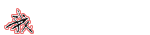讲完了滤波器实现滤波的过程。也在课堂上演示了低通滤波器、高通滤波器、带通滤波器的设计过程以及滤波过程，所以本堂课就要来梳理一下滤波器的种类。类型就是刚才提到的低通、高通、带通，还有个带阻。但种类就只有IIR和FIR两种。之前演示的程序都只是涉及FIR滤波器，现在要开始和IIR滤波器打交道了。大家知道这两者的区别吗？开始讲解了！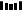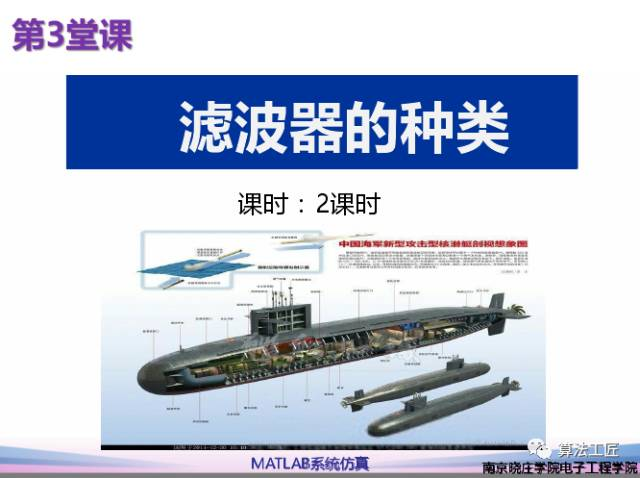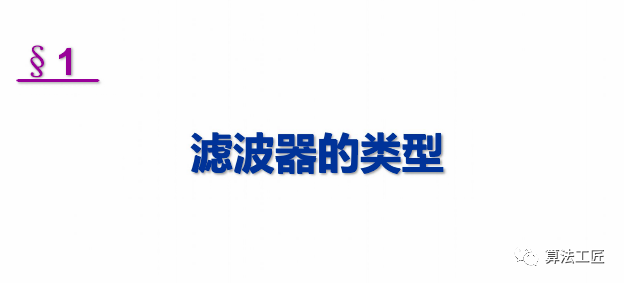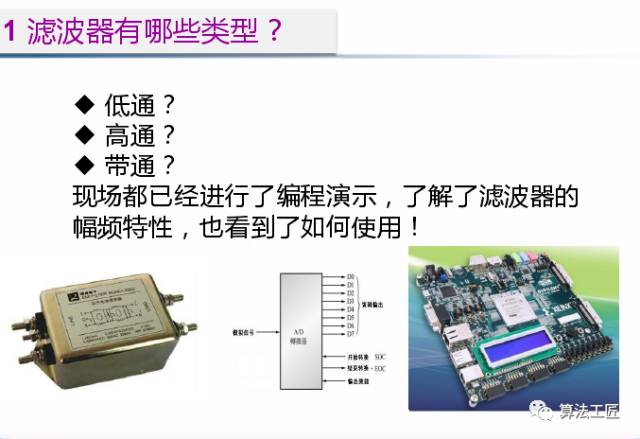带阻滤波器不怎么常用，因此没有列出！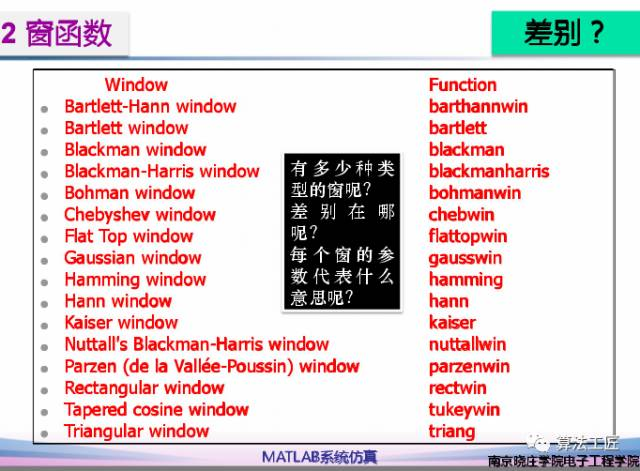本图几乎列出了所有的窗函数！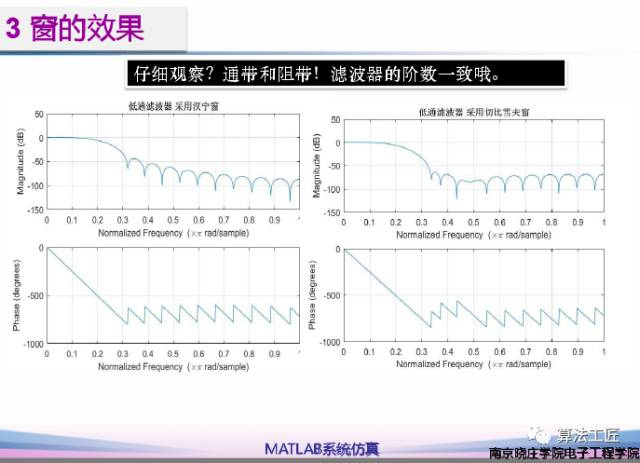在课堂上，演示了三种窗。汉明窗、切比雪夫窗、凯泽窗！差别还记得吗？记不住，就看图说话！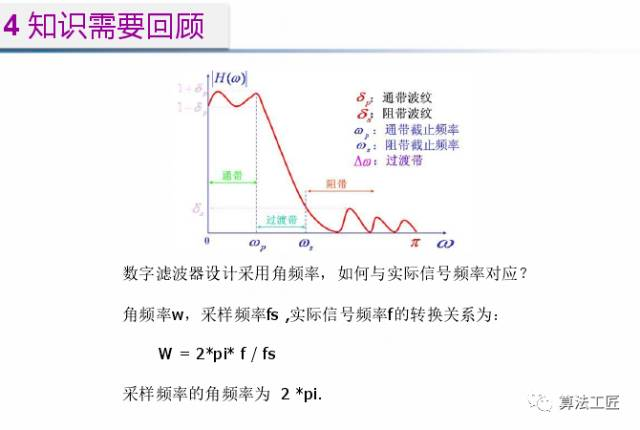正式开始讲解IIR滤波器！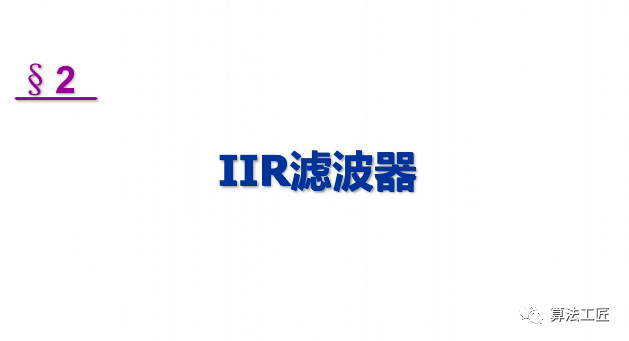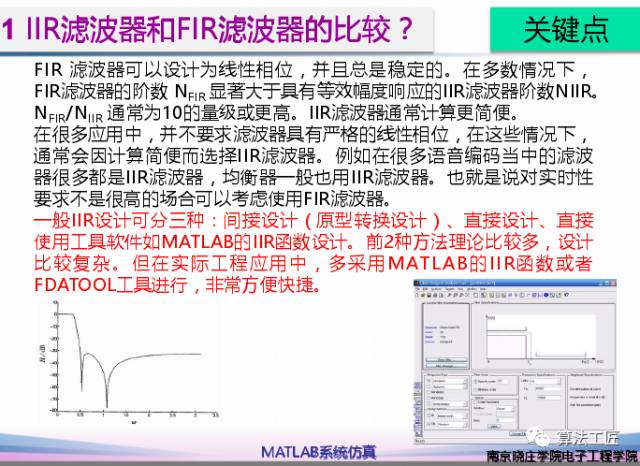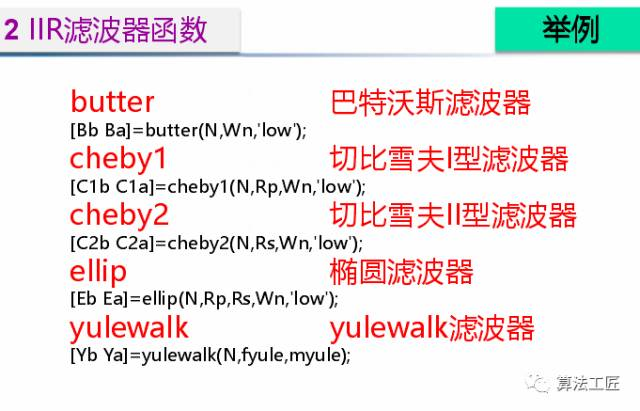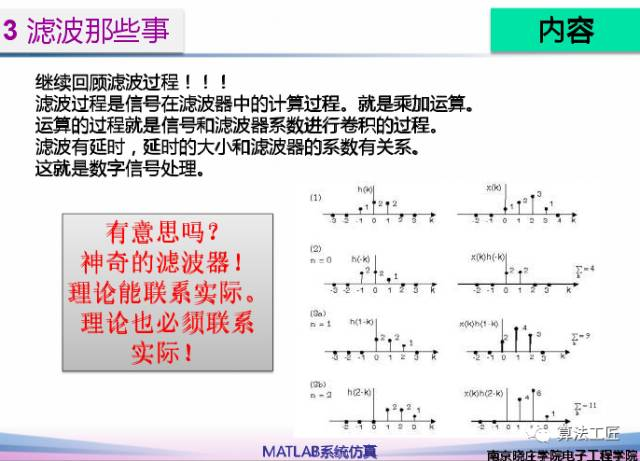本人在之前的工作过程中很少使用IIR滤波器，如果在滤波一般的频率信号时，不考虑相位群延迟的影响，IIR滤波器确实可以使用，而且占用资源也少。但是对于宽带信号，含有很多种频率分量，如果滤波器没有线性相位的特性，那么滤波后将出现很多问题。因此，在通信信号的数字处理过程中，很少使用。未完，待续！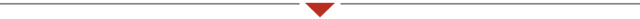题外话！看过一本柴静的书叫《看见》，过了一两年，她的一个涉及环保的节目出来了，火了，搞的环保题材的股票都在涨，可见影响力之大。于是引起很多的争论。那2017年5月的股市如何呢？看图吧！不想多讲，全是痛！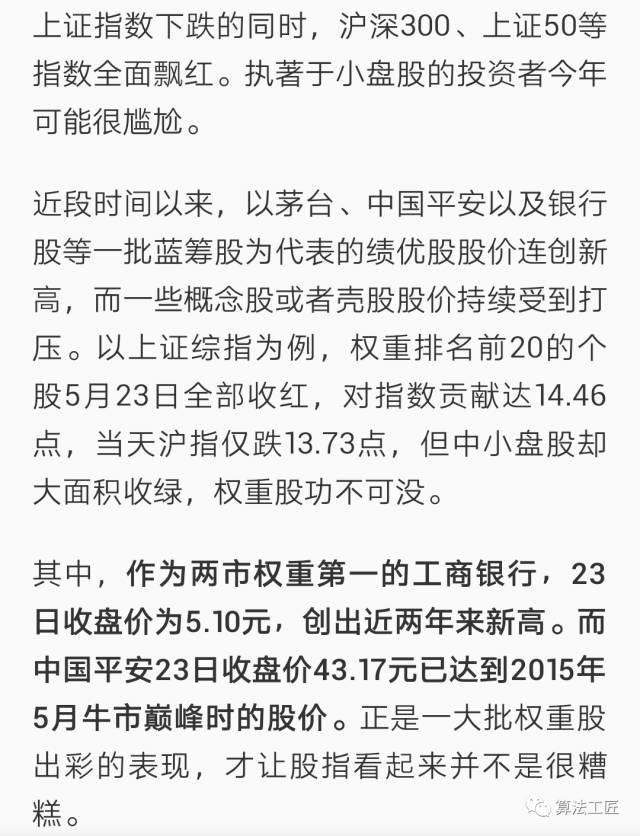等是硬道理！2020年，带痛总结！修订记录20170117 完成初稿；20170329 增加程序；20170419 修订内容；20201007 修订文字；点击“凡事小小说：出租屋的生活！凡事小小说：衣服没变，人变了！凡事小小说：不要也不能活在别人的评价里！电气信息类专业课程之matlab系统仿真 第一章 信号和滤波器电气信息类专业课程之matlab系统仿真 第二章 深入理解滤波器？


展开全文matlab 滤波
• 5.2.2 巴特沃斯低通逼近 例:设计一个模拟低通巴特沃斯...即可得到所求的系统函数 得系统函数 2也可先求极点再构造系统函数 系统函数的4个极点为 5.2.4 模拟高通带通带阻滤波器设计 模拟高通带通及带阻滤波器的系统函数
• 列写无源低通、高通、带通和带阻滤波器的网络函数。 2． 用示波器观察二阶无源滤波器的幅频特性曲线。三、实验仪器1． 信号与系统实验箱 一台 2． 信号系统实验平台3． 二阶无源滤波器模块(DYT3000-61) 一块 4...
二阶无源滤波器一、实验目的1． 了解RC 无源滤波器的种类、基本结构及其特性。 2． 学会列写无源滤波器网络函数的方法。 3． 学会测量无源滤波器幅频特性的方法。二、实验内容1． 列写无源低通、高通、带通和带阻滤波器的网络函数。 2． 用示波器观察二阶无源滤波器的幅频特性曲线。三、实验仪器1． 信号与系统实验箱 一台 2． 信号系统实验平台3． 二阶无源滤波器模块(DYT3000-61) 一块 4． 20MHz 双踪示波器 一台 5． 连接线若干四、实验原理滤波器是一种能使有用频率信号通过而同时抑制(或大为衰减)无用频率信号的电子装置。工程上常用它作信号处理、数据传送和抑制干扰等。这里主要讨论模拟滤波器。1. 基本概念及初步定义滤波器的一般结构如图17-1所示。图中的V i (t )表示输入信号，V o (t )为输出信号。假设滤波器是一个线性时不变网络，则在复频域内其传递函数(系统函数)为()()()o i V s A s V s图17-1 滤波电路的一般结构式中A (s )是滤波电路的电压传递函数，一般为复数。对于频率来说(s ＝j ω)则有
展开全文• 巴特沃思、切比雪夫模拟低通滤波器通常是设计模拟高通、带通和带阻滤波器的原型,先按给定频率响应巴特沃思、切比雪夫低通Ha(s)逼近,然后由选定Ha(s)实现二端口网络的电路结构和参数值。在此对达林顿T型和П型电路...
• 2.2.2低通原型滤波器的系统函数 20 2.2.3 椭圆滤波器及最小阶数的选择 21 2.2.4贝塞尔滤波器 22 第3章 IIR数字滤波器的设计 23 3.1 IIR数字滤波器的设计方法 23 3.2 IIR滤波器经典设计 24 3.3 IIR滤波器直接设计 33 ...
• 按频率特性分类：高通、低通、带通和带阻； 按冲激响应特性分类： FIR滤波器：有限冲激响应，滤波器的输出只与当前输入和有限历史输入有关，无反馈回路，不存在不稳定的问题，其传递函数只有零点，这种滤波器的冲....自动控制原理
• ' 调用 Fz_LP 子程序， 将低通模拟滤波器的转移函数变量 s 映射为低通数字滤波器的系统函数变量 z Call Fz_LP(F1(), F2(), ord_t) ' End If '''''''''''''''''''' If PbType = 1 Then ' 高通滤波器 ; ...
• 集总低通原型滤波器是现代网络综合法设计滤波器的基础，各种低通、高通、带通、带阻滤波器大都是根据此特性推导出来的。正因如此，才使得滤波器的设计得以简化，精度得以提高。 　理想的低通滤波器应该能使所有低于...
• 例如：按频率选择特性可以分为：低通、高通、带通、带阻滤波器等；按实现方式可以分为：LC滤波器、声表面波/体声波滤波器、螺旋滤波器、介质滤波器、腔体滤波器、高温超导滤波器、平面结构滤波器。按不同频率...
• 系统函数决定了滤波器系统 分类 滤波能力 低通；高通；带通；带阻 输入信号 数字；模拟 滤波阶数 一阶；二阶；三阶；三阶以上 滤波特性 有源：用到有源器件：R,L,C 无源：放大器 性能指标 通带边缘频率 Pass ...